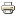## Error message

Deprecated function: Array and string offset access syntax with curly braces is deprecated in include_once() (line 20 of /mnt/web104/a0/07/510451407/htdocs/includes/file.phar.inc).

# The Four-in-a Tree Problem in Triangle-Free GraphsWorking paper
Author/s:
Nicolas Derhy, Christophe Picouleau and Nicolas Trotignon
Issue number:
2008.23
Publisher:
Centre d’Economie de la Sorbonne
Year:
2008
The three-in-a-tree algorithm of Chudnovsky and Seymour decides in time O(n4) whether three given vertices of a graph belong to an induced tree. Here, we study four- in-a-tree for triangle-free graphs. We give a structural answer to the following question: how does look like a triangle-free graph such that no induced tree covers four given vertices ? Our main result says that any such graph must have the “same structure”, in a sense to be deﬁned precisely, as a square or a cube. We provide an O(nm)-time algorithm that given a triangle-free graph G together with four vertices outputs either an induced tree that contains them or a partition of V (G) certifying that no such tree exists. We prove that the problem of deciding whether there exists a tree T covering the four vertices such that at most one vertex of T has degree at least 3 is NP-complete.
Developed by Paolo Gittoi